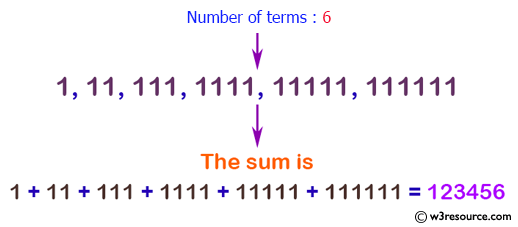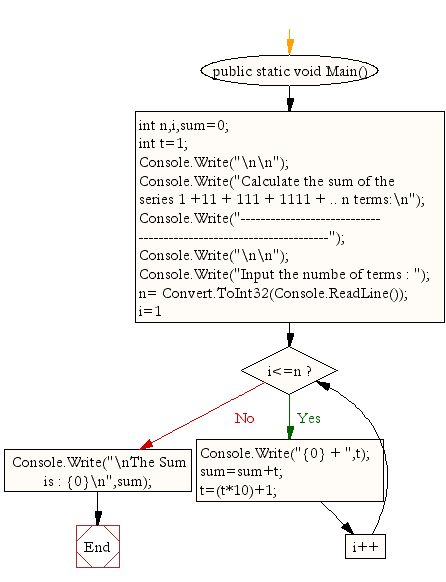﻿ C# - Calculate the sum of the series 1+11+111+ 1111+ .. N# C# Sharp Exercises: Calculate the sum of the series 1 +11 + 111 + 1111 + .. n terms

## C# Sharp For Loop: Exercise-26 with Solution

Write a program in C# Sharp to find the sum of the series 1 +11 + 111 + 1111 + .. n terms.

Pictorial Presentation:Sample Solution:

C# Sharp Code:

``````using System;
public class Exercise26
{
public static void Main()
{
int n,i,sum=0;
int t=1;

Console.Write("\n\n");
Console.Write("Calculate the sum of the series 1 +11 + 111 + 1111 + .. n terms:\n");
Console.Write("------------------------------------------------------------------");
Console.Write("\n\n");

Console.Write("Input the numbe of terms : ");
for(i=1;i<=n;i++)
{
Console.Write("{0} + ",t);
sum=sum+t;
t=(t*10)+1;
}
Console.Write("\nThe Sum is : {0}\n",sum);
}
}
```
```

Sample Output:

```Calculate the sum of the series 1 +11 + 111 + 1111 + .. n terms:
------------------------------------------------------------------
Input the numbe of terms : 6
1 + 11 + 111 + 1111 + 11111 + 111111 +
The Sum is : 123456
```

Flowchart:C# Sharp Code Editor:

Contribute your code and comments through Disqus.

What is the difficulty level of this exercise?

Test your Programming skills with w3resource's quiz.

﻿